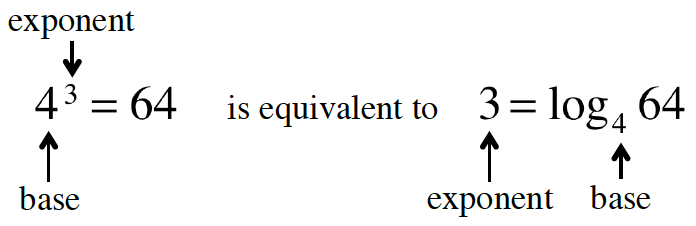### Home > CCA2 > Chapter Ch5 > Lesson 5.2.2 > Problem5-74

5-74.

Every exponential equation has an equivalent logarithmic form and every logarithmic equation has an equivalent exponential form. For example,Copy the table shown below and fill in the missing form in each row.

 Exponential Form Logarithmic Form a. $y=5^x$ b. $y=\log_7\left(x\right)$ c. $8^x=y$ d. $\text{A}^\text{K} = \text{C}$ e. K = log$_\text{A}$(C) f. log$_{1/2}$(K) = N

 Exponential Form Logarithmic Form a. $y=5^x$ ${\color{red}{x=\log_5\left(y\right)}}$ b. ${\color{red}{x=7^x}}$ $y=\log_7\left(x\right)$ c. $8^x=y$ ${\color{red}{x=\log_8\left(y\right)}}$ d. $\text{A}^\text{K} = \text{C}$ ${\color{red}{K=\log_A\left(C\right)}}$ e. ${\color{red}{C=A^K}}$ K = log$_\text{A}$(C) f. ${\color{red}{K=({\frac{1}{2}})^N}}$ log$_{1/2}$(K) = N